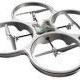ROS Resources: Documentation | Support | Discussion Forum | Index | Service Status | Q&A answers.ros.org

# How to display CV_32FC2 images in ROSI'm trying to use OpenCV to calculate real-rime optical flow and display it in ROS. The task, using provided OpenCV's functionality, seems fairly easy. I've used uvc_camera and cv_bridge to capture images with ROS and convert them to cv::Mat. Then, I'm using calcOpticalFlowFarneback to calculate optical flow, which returns a cv::Mat optical flow image of type CV_32FC2.

The code I'm using to publish the image looks like this:

cv_bridge::CvImage flowImage;
sensor_msgs::ImagePtr flowImagePtr;

if (!_flow.empty()) {
flowImage.encoding = sensor_msgs::image_encodings::TYPE_32FC2;
flowImage.image = _flow; //_flow is calculated elsewhere

flowImagePtr = flowImage.toImageMsg();
publishCvImage(flowImagePtr); //uses a publisher to publish image
}


The code works fine, but ROS is unable to display floating point images (I tried either image_view and rviz). What is the right way to convert those images to a displayable form?

Cheers, Tom.

Edit: Eventually I'm using these two methods to visualize the results (the below code bases on this):

cvImagePtr = cv_bridge::toCvCopy(image, sensor_msgs::image_encodings::RGB8);
flowImage = cvImagePtr->image; //original RGB captured image, needs to be converted to MONO8 for cv::calcOpticalFlowFarneback
cv::Mat velx = cv::Mat(flowImage.size(), CV_32FC1);
cv::Mat vely = cv::Mat(flowImage.size(), CV_32FC1);
cv::Mat flowMatrix; //outcome of cv::calcOpticalFlowFarneback

void OpticalFlow::extractFlowFieldFarneback() {

for (int row = 0; row < flowMatrix.rows; row++) {
float *vx = (float*)(velx.data + velx.step * row);
float *vy = (float*)(vely.data + vely.step * row);

const float* f = (const float*)(flowMatrix.data + flowMatrix.step * row);
for (int col = 0; col < flowMatrix.cols; col++) {
vx[col] = f[2*col];
vy[col] = f[2*col+1];
}
}
}

void OpticalFlow::overlayFlowFieldFarneback() {

if (!velx.empty() && !vely.empty()) {

for (int row = 0; row < flowImage.rows; row++) {
float *vx = (float*)(velx.data + velx.step * row);
float *vy = (float*)(vely.data + vely.step * row);

for (int col = 0; col < flowImage.cols; col++) {
double length = sqrt(vx[col]*vx[col] + vy[col]*vy[col]);

if (length > 1) {
cv::Point2i p1(col, row);
cv::Point2i p2(col + (int)vx[col], row + (int)vy[col]);

cv::line(flowImage, p1, p2, cvScalar(255, 0, 0, 0));
}
}
}
}
}

edit retag close merge delete

Sort by » oldest newest most votedIf you just want to visually inspect it, you could scale the value range according to the maximum value:

00436 void depthToCV8UC1(const cv::Mat& float_img, cv::Mat& mono8_img){
00437   //Process images
00438   if(mono8_img.rows != float_img.rows || mono8_img.cols != float_img.cols){
00439     mono8_img = cv::Mat(float_img.size(), CV_8UC1);}
00440   //The following doesn't work if there are NaNs
00441   double minVal, maxVal;
00442   minMaxLoc(float_img, &minVal, &maxVal);
00443   ROS_DEBUG("Minimum/Maximum Depth in current image: %f/%f", minVal, maxVal);
00446   cv::convertScaleAbs(float_img, mono8_img, 100, 0.0);
00447 }

more

Thanks Felix, the idea is ok, but I get the error: OpenCV Error: Assertion failed (img.channels() == 1) in minMaxLoc. What I'd like to achieve the most, is to obtain nice real-time images with arrows showing temporary optical-flow vectors overlayed over the image - is there a simple way?
Ah, that is because you have channels for x and y. I don't know any simple way to get that visualized, sorry

See drawOptFlowMap at OpenCV.org

more